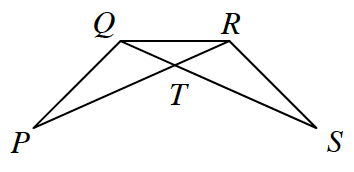### Home > CCG > Chapter Ch8 > Lesson 8.1.3 > Problem8-30

8-30.

In the figure below, if $PQ = SR$ and $PR = SQ$, prove that $\angle P \cong \angle S$. Write your proof either in a flowchart or in two-column proof form.Statement Reason
$PR = SQ$ Given
$PQ = RS$ Given
$QR = QR$ Reflexive
$\Delta PQR \cong \Delta SRQ \text{ SSS}\cong$
$\angle P \cong \angle S \cong \Delta \rightarrow \: \cong \text{ parts}$.

Be sure to look at the reasoning and understand why this proof is organized as it is.